## Marco's Web Center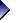Menu for BooksDelphi 2007 HandbookMastering Delphi 2005Essential Delphi 8 for .NETMastering Delphi 7Essential PascalEssential DelphiBuy Books OnlineMarco's TechBookStoreSite MenuObject Pascal HandbookDelphi Handbooks CollectionMastering Borland Delphi 2005(Old) White Papers(Old)Tools(Old) ConferencesMy Other SitesItalian Site (www.marcocantu.it)the delphi searchAdvertisingHome My Blog Books Object Pascal MarcoThe cover of the 4th edition of Essential Pascal, the first available in print (and PDF) on Lulu.com.

# Chapter 3 Types, Variables, and Constants

The original Pascal language was based on some simple notions, which have now become quite common in programming languages. The first is the notion of data type. The type determines the values a variable can have, and the operations that can be performed on it. The concept of type is stronger in Pascal than in C, where the arithmetic data types are almost interchangeable, and much stronger than in the original versions of BASIC, which had no similar concept.

## Variables

Pascal requires all variables to be declared before they are used. Every time you declare a variable, you must specify a data type. Here are some sample variable declarations:

```var
Value: Integer;
IsCorrect: Boolean;
A, B: Char;
```

The var keyword can be used in several places in the code, such as at the beginning of the code of a function or procedure, to declare variables local to the routine, or inside a unit to declare global variables. After the var keyword comes a list of variable names, followed by a colon and the name of the data type. You can write more than one variable name on a single line, as in the last statement above.

Once you have defined a variable of a given type, you can perform on it only the operations supported by its data type. For example, you can use the Boolean value in a test and the integer value in a numerical expression. You cannot mix Booleans and integers (as you can with the C language).

Using simple assignments, we can write the following code:

```Value := 10;
IsCorrect := True;
```

But the next statement is not correct, because the two variables have different data types:

```Value := IsCorrect; // error
```

If you try to compile this code, Delphi issues a compiler error with this description: Incompatible types: 'Integer' and 'Boolean'. Usually, errors like this are programming errors, because it does not make sense to assign a True or False value to a variable of the Integer data type. You should not blame Delphi for these errors. It only warns you that there is something wrong in the code.

Of course, it is often possible to convert the value of a variable from one type into a different type. In some cases, this conversion is automatic, but usually you need to call a specific system function that changes the internal representation of the data.

In Delphi you can assign an initial value to a global variable while you declare it. For example, you can write:

```var
Value: Integer = 10;
Correct: Boolean = True;
```

This initialization technique works only for global variables, not for variables declared inside the scope of a procedure or method.

## Constants

Pascal also allows the declaration of constants to name values that do not change during program execution. To declare a constant you don't need to specify a data type, but only assign an initial value. The compiler will look at the value and automatically use its proper data type. Here are some sample declarations:

```const
Thousand = 1000;
Pi = 3.14;
AuthorName = 'Marco Cantù';
```

Delphi determines the constant's data type based on its value. In the example above, the Thousand constant is assumed to be of type SmallInt, the smallest integral type which can hold it. If you want to tell Delphi to use a specific type you can simply add the type name in the declaration, as in:

```const
Thousand: Integer = 1000;
```

When you declare a constant, the compiler can choose whether to assign a memory location to the constant, and save its value there, or to duplicate the actual value each time the constant is used. This second approach makes sense particularly for simple constants.

Note: The 16-bit version of Delphi allows you to change the value of a typed constant at run-time, as if it was a variable. The 32-bit version still permits this behavior for backward compatibility when you enable the \$J compiler directive, or use the corresponding Assignable typed constants check box of the Compiler page of the Project Options dialog box. Although this is the default, you are strongly advised not to use this trick as a general programming technique. Assigning a new value to a constant disables all the compiler optimizations on constants. In such a case, simply declare a variable, instead.

## Resource String Constants

When you define a string constant, instead of writing:

```const
AuthorName = 'Marco Cantù';
```

starting with Delphi 3 you can write the following:

```resourcestring
AuthorName = 'Marco Cantù';
```

In both cases you are defining a constant; that is, a value you don't change during program execution. The difference is only in the implementation. A string constant defined with the resourcestring directive is stored in the resources of the program, in a string table.

To see this capability in action, you can look at the ResStr example, which has a button with the following code:

```resourcestring
AuthorName = 'Marco Cantù';
BookName = 'Essential Pascal';

procedure TForm1.Button1Click(Sender: TObject);
begin
ShowMessage (BookName + #13 + AuthorName);
end;
```

The output of the two strings appears on separate lines because the strings are separated by the newline character (indicated by its numerical value in the #13 character-type constant).

The interesting aspect of this program is that if you examine it with a resource explorer (there is one available among the examples that ship with Delphi) you'll see the new strings in the resources. This means that the strings are not part of the compiled code but stored in a separate area of the executable file (the EXE file).

Note: In short, the advantage of resources is in an efficient memory handling performed by Windows and in the possibility of localizing a program (translating the strings to a different language) without having to modify its source code.

## Data Types

In Pascal there are several predefined data types, which can be divided into three groups: ordinal types, real types, and strings. We'll discuss ordinal and real types in the following sections, while strings are covered later in this chapter. In this section I'll also introduce some types defined by the Delphi libraries (not predefined by the compiler), which can be considered predefined types.

Delphi also includes a non-typed data type, called variant, and discussed in Chapter 10 of this book. Strangely enough a variant is a type without proper type-checking. It was introduced in Delphi 2 to handle OLE Automation.

## Ordinal Types

Ordinal types are based on the concept of order or sequence. Not only can you compare two values to see which is higher, but you can also ask for the value following or preceding a given value or compute the lowest or highest possible value.

The three most important predefined ordinal types are Integer, Boolean, and Char (character). However, there are a number of other related types that have the same meaning but a different internal representation and range of values. The following Table 3.1 lists the ordinal data types used for representing numbers.

Table 3.1: Ordinal data types for numbers

Size Signed
Range
Unsigned
Range
8 bits ShortInt
-128 to 127
Byte
0 to 255
16 bits SmallInt
-32768 to 32767
Word
0 to 65,535
32 bits LongInt
-2,147,483,648 to 2,147,483,647
LongWord (since Delphi 4)
0 to 4,294,967,295
64 bits Int64
16/32 bits Integer Cardinal

As you can see, these types correspond to different representations of numbers, depending on the number of bits used to express the value, and the presence or absence of a sign bit. Signed values can be positive or negative, but have a smaller range of values, because one less bit is available for the value itself. You can refer to the Range example, discussed in the next section, for the actual range of values of each type.

The last group (marked as 16/32) indicates values having a different representation in the 16-bit and 32-bit versions of Delphi. Integer and Cardinal are frequently used, because they correspond to the native representation of numbers in the CPU.

### Integral Types in Delphi 4

In Delphi 3, the 32-bit unsigned numbers indicated by the Cardinal type were actually 31-bit values, with a range up to 2 gigabytes. Delphi 4 introduced a new unsigned numeric type, LongWord, which uses a truly 32-bit value up to 4 gigabytes. The Cardinal type is now an alias of the new LongWord type. LongWord permits 2GB more data to be addressed by an unsigned number, as mentioned above. Moreover, it corresponds to the native representation of numbers in the CPU.

Another new type introduced in Delphi 4 is the Int64 type, which represents integer numbers with up to 18 digits. This new type is fully supported by some of the ordinal type routines (such as High and Low), numeric routines (such as Inc and Dec), and string-conversion routines (such as IntToStr). For the opposite conversion, from a string to a number, there are two new specific functions: StrToInt64 and StrToInt64Def.

### Boolean

Boolean values other than the Boolean type are seldom used. Some Boolean values with specific representations are required by Windows API functions. The types are ByteBool, WordBool, and LongBool.

In Delphi 3 for compatibility with Visual Basic and OLE automation, the data types ByteBool, WordBool, and LongBool were modified to represent the value True with -1, while the value False is still 0. The Boolean data type remains unchanged (True is 1, False is 0). If you've used explicit typecasts in your Delphi 2 code, porting the code to later versions of Delphi might result in errors.

### Characters

Finally there are two different representation for characters: ANSIChar and WideChar. The first type represents 8-bit characters, corresponding to the ANSI character set traditionally used by Windows; the second represents 16-bit characters, corresponding to the new Unicode characters supported by Windows NT, and only partially by Windows 95 and 98. Most of the time you'll simply use the Char type, which in Delphi 3 corresponds to ANSIChar. Keep in mind, anyway, that the first 256 Unicode characters correspond exactly to the ANSI characters.

Constant characters can be represented with their symbolic notation, as in 'k', or with a numeric notation, as in #78. The latter can also be expressed using the Chr function, as in Chr (78). The opposite conversion can be done with the Ord function.

It is generally better to use the symbolic notation when indicating letters, digits, or symbols. When referring to special characters, instead, you'll generally use the numeric notation. The following list includes some of the most commonly used special characters:

• #9 tabulator
• #10 newline
• #13 carriage return (enter key)

### The Range Example

To give you an idea of the different ranges of some of the ordinal types, I've written a simple Delphi program named Range. Some results are shown in Figure 3.1.

FIGURE 3.1: The Range example displays some information about ordinal data types (Integers in this case).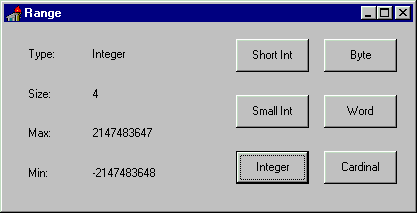The Range program is based on a simple form, which has six buttons (each named after an ordinal data type) and some labels for categories of information, as you can see in Figure 3.1. Some of the labels are used to hold static text, others to show the information about the type each time one of the buttons is pressed.

Every time you press one of the buttons on the right, the program updates the labels with the output. Different labels show the data type, number of bytes used, and the maximum and minimum values the data type can store. Each button has its own OnClick event-response method because the code used to compute the three values is slightly different from button to button. For example, here is the source code of the OnClick event for the Integer button (BtnInteger):

```procedure TFormRange.BtnIntegerClick(Sender: TObject);
begin
LabelType.Caption := 'Integer';
LabelSize.Caption := IntToStr (SizeOf (Integer));
LabelMax.Caption := IntToStr (High (Integer));
LabelMin.Caption := IntToStr (Low (Integer));
end;
```

If you have some experience with Delphi programming, you can examine the source code of the program to understand how it works. For beginners, it's enough to note the use of three functions: SizeOf, High, and Low. The results of the last two functions are ordinals of the same kind (in this case, integers), and the result of the SizeOf function is always an integer. The return value of each of these functions is first translated into strings using the IntToStr function, then copied to the captions of the three labels.

The methods associated with the other buttons are very similar to the one above. The only real difference is in the data type passed as a parameter to the various functions. Figure 3.2 shows the result of executing this same program under Windows 95 after it has been recompiled with the 16-bit version of Delphi. Comparing Figure 3.1 with Figure 3.2, you can see the difference between the 16-bit and 32-bit Integer data types.

FIGURE 3.2: The output of the 16-bit version of the Range example, again showing information about integers.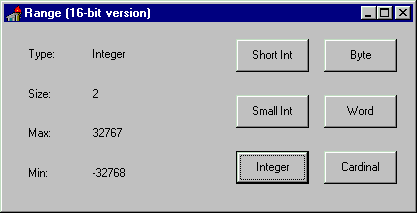The size of the Integer type varies depending on the CPU and operating system you are using. In 16-bit Windows, an Integer variable is two bytes wide. In 32-bit Windows, an Integer is four bytes wide. For this reason, when you recompile the Range example, you get a different output.

The two different representations of the Integer type are not a problem, as long as your program doesn't make any assumptions about the size of integers. If you happen to save an Integer to a file using one version and retrieve it with another, though, you're going to have some trouble. In this situation, you should choose a platform-independent data type (such as LongInt or SmallInt). For mathematical computation or generic code, your best bet is to stick with the standard integral representation for the specific platform--that is, use the Integer type--because this is what the CPU likes best. The Integer type should be your first choice when handling integer numbers. Use a different representation only when there is a compelling reason to do so.

### Ordinal Types Routines

There are some system routines (routines defined in the Pascal language and in the Delphi system unit) that work on ordinal types. They are shown in Table 3.2. C++ programmers should notice that the two versions of the Inc procedure, with one or two parameters, correspond to the ++ and += operators (the same holds for the Dec procedure).

Table 3.2: System Routines for Ordinal Types

RoutinePurpose
DecDecrements the variable passed as parameter, by one or by the value of the optional second parameter.
IncIncrements the variable passed as parameter, by one or by the specified value.
OddReturns True if the argument is an odd number.
PredReturns the value before the argument in the order determined by the data type, the predecessor.
SuccReturns the value after the argument, the successor.
OrdReturns a number indicating the order of the argument within the set of values of the data type.
LowReturns the lowest value in the range of the ordinal type passed as its parameter.
HighReturns the highest value in the range of the ordinal data type.

Notice that some of these routines, when applied to constants, are automatically evaluated by the compiler and replaced by their value. For example if you call High(X) where X is defined as an Integer, the compiler can simply replace the expression with the highest possible value of the Integer data type.

## Real Types

Real types represent floating-point numbers in various formats. The smallest storage size is given by Single numbers, which are implemented with a 4-byte value. Then there are Double floating-point numbers, implemented with 8 bytes, and Extended numbers, implemented with 10 bytes. These are all floating-point data types with different precision, which correspond to the IEEE standard floating-point representations, and are directly supported by the CPU numeric coprocessor, for maximum speed.

In Delphi 2 and Delphi 3 the Real type had the same definition as in the 16-bit version; it was a 48-bit type. But its usage was deprecated by Borland, which suggested that you use the Single, Double, and Extended types instead. The reason for their suggestion is that the old 6-byte format is neither supported by the Intel CPU nor listed among the official IEEE real types. To completely overcome the problem, Delphi 4 modifies the definition of the Real type to represent a standard 8-byte (64-bit) floating-point number.

In addition to the advantage of using a standard definition, this change allows components to publish properties based on the Real type, something Delphi 3 did not allow. Among the disadvantages there might be compatibility problems. If necessary, you can overcome the possibility of incompatibility by sticking to the Delphi 2 and 3 definition of the type; do this by using the following compiler option:

```{\$REALCOMPATIBILITY ON}
```

There are also two strange data types: Comp describes very big integers using 8 bytes (which can hold numbers with 18 decimal digits); and Currency (not available in 16-bit Delphi) indicates a fixed-point decimal value with four decimal digits, and the same 64-bit representation as the Comp type. As the name implies, the Currency data type has been added to handle very precise monetary values, with four decimal places.

We cannot build a program similar to the Range example with real data types, because we cannot use the High and Low functions or the Ord function on real-type variables. Real types represent (in theory) an infinite set of numbers; ordinal types represent a fixed set of values.

Note: Let me explain this better. when you have the integer 23 you can determine which is the following value. Integers are finite (they have a determined range and they have an order). Floating point numbers are infinite even within a small range, and have no order: in fact, how many values are there between 23 and 24? And which number follows 23.46? It is 23.47, 23.461, or 23.4601? That's really hard to know!

For this reason, it makes sense to ask for the ordinal position of the character w in the range of the Char data type, but it makes no sense at all to ask the same question about 7143.1562 in the range of a floating-point data type. Although you can indeed know whether one real number has a higher value than another, it makes no sense to ask how many real numbers exist before a given number (this is the meaning of the Ord function).

Real types have a limited role in the user interface portion of the code (the Windows side), but they are fully supported by Delphi, including the database side. The support of IEEE standard floating-point types makes the Object Pascal language completely appropriate for the wide range of programs that require numerical computations. If you are interested in this aspect, you can look at the arithmetic functions provided by Delphi in the system unit (see the Delphi Help for more details).

Note: Delphi also has a Math unit that defines advanced mathematical routines, covering trigonometric functions (such as the ArcCosh function), finance (such as the InterestPayment function), and statistics (such as the MeanAndStdDev procedure). There are a number of these routines, some of which sound quite strange to me, such as the MomentSkewKurtosis procedure (I'll let you find out what this is).

## Date and Time

Delphi uses real types also to handle date and time information. To be more precise Delphi defines a specific TDateTime data type. This is a floating-point type, because the type must be wide enough to store years, months, days, hours, minutes, and seconds, down to millisecond resolution in a single variable. Dates are stored as the number of days since 1899-12-30 (with negative values indicating dates before 1899) in the integer part of the TDateTime value. Times are stored as fractions of a day in the decimal part of the value.

TDateTime is not a predefined type the compiler understands, but it is defined in the system unit as:

```type
TDateTime = type Double;
```

Using the TDateTime type is quite easy, because Delphi includes a number of functions that operate on this type. You can find a list of these functions in Table 3.3.

Table 3.3: System Routines for the TDateTime Type

RoutineDescription
NowReturns the current date and time into a single TDateTime value.
DateReturns only the current date.
TimeReturns only the current time.
DateTimeToStrConverts a date and time value into a string, using default formatting; to have more control on the conversion use the FormatDateTime function instead.
DateTimeToStringCopies the date and time values into a string buffer, with default formatting.
DateToStrConverts the date portion of a TDateTime value into a string.
TimeToStrConverts the time portion of a TDateTime value into a string.
FormatDateTimeFormats a date and time using the specified format; you can specify which values you want to see and which format to use, providing a complex format string.
StrToDateTimeConverts a string with date and time information to a TDateTime value, raising an exception in case of an error in the format of the string.
StrToDateConverts a string with a date value into the TDateTime format.
StrToTimeConverts a string with a time value into the TDateTime format.
DayOfWeekReturns the number corresponding to the day of the week of the TDateTime value passed as parameter.
DecodeDateRetrieves the year, month, and day values from a date value.
DecodeTimeRetrieves out of a time value.
EncodeDateTurns year, month, and day values into a TDateTime value.
EncodeTimeTurns hour, minute, second, and millisecond values into a TDateTime value.

To show you how to use this data type and some of its related routines, I've built a simple example, named TimeNow. The main form of this example has a Button and a ListBox component. When the program starts it automatically computes and displays the current time and date. Every time the button is pressed, the program shows the time elapsed since the program started.

Here is the code related to the OnCreate event of the form:

```procedure TFormTimeNow.FormCreate(Sender: TObject);
begin
StartTime := Now;
ListBox1.Items.Add ('Press button for elapsed time');
end;
```

The first statement is a call to the Now function, which returns the current date and time. This value is stored in the StartTime variable, declared as a global variable as follows:

```var
FormTimeNow: TFormTimeNow;
StartTime: TDateTime;
```

I've added only the second declaration, since the first is provided by Delphi. By default, it is the following:

```var
Form1: TForm1;
```

Changing the name of the form, this declaration is automatically updated. Using global variables is actually not the best approach: It should be better to use a private field of the form class, a topic related to object-oriented programming and discussed in Mastering Delphi 4.

The next three statements add three items to the ListBox component on the left of the form, with the result you can see in Figure 3.3. The first line contains the time portion of the TDateTime value converted into a string, the second the date portion of the same value. At the end the code adds a simple reminder.

FIGURE 3.3: The output of the TimeNow example at startup.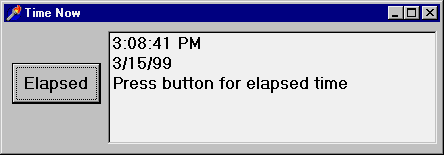This third string is replaced by the program when the user clicks on the Elapsed button:

```procedure TFormTimeNow.ButtonElapsedClick(Sender: TObject);
var
StopTime: TDateTime;
begin
StopTime := Now;
ListBox1.Items  :=  FormatDateTime ('hh:nn:ss',
StopTime - StartTime);
end;
```

This code retrieves the new time and computes the difference from the time value stored when the program started. Because we need to use a value that we computed in a different event handler, we had to store it in a global variable. There are actually better alternatives, based on classes.

Note: The code that replaces the current value of the third string uses the index 2. The reason is that the items of a list box are zero-based: the first item is number 0, the second number 1, and the third number 2. More on this as we cover arrays.

Besides calling TimeToStr and DateToStr you can use the more powerful FormatDateTime function, as I've done in the last method above (see the Delphi Help file for details on the formatting parameters). Notice also that time and date values are transformed into strings depending on Windows international settings. Delphi reads these values from the system, and copies them to a number of global constants declared in the SysUtils unit. Some of them are:

```DateSeparator: Char;
ShortDateFormat: string;
LongDateFormat: string;
TimeSeparator: Char;
TimeAMString: string;
TimePMString: string;
ShortTimeFormat: string;
LongTimeFormat: string;
ShortMonthNames: array [1..12] of string;
LongMonthNames: array [1..12] of string;
ShortDayNames: array [1..7] of string;
LongDayNames: array [1..7] of string;
```

More global constants relate to currency and floating-point number formatting. You can find the complete list in the Delphi Help file under the topic Currency and date/time formatting variables.

Note: Delphi includes a DateTimePicker component, which provides a sophisticated way to input a date, selecting it from a calendar.

## Specific Windows Types

The predefined data types we have seen so far are part of the Pascal language. Delphi also includes other data types defined by Windows. These data types are not an integral part of the language, but they are part of the Windows libraries. Windows types include new default types (such as DWORD or UINT), many records (or structures), several pointer types, and so on.

Among Windows data types, the most important type is represented by handles, discussed in Chapter 9.

## Typecasting and Type Conversions

As we have seen, you cannot assign a variable to another one of a different type. In case you need to do this, there are two choices. The first choice is typecasting, which uses a simple functional notation, with the name of the destination data type:

```var
N: Integer;
C: Char;
B: Boolean;
begin
N := Integer ('X');
C := Char (N);
B := Boolean (0);
```

You can typecast between data types having the same size. It is usually safe to typecast between ordinal types, or between real types, but you can also typecast between pointer types (and also objects) as long as you know what you are doing.

Casting, however, is generally a dangerous programming practice, because it allows you to access a value as if it represented something else. Since the internal representations of data types generally do not match, you risk hard-to-track errors. For this reason, you should generally avoid typecasting.

The second choice is to use a type-conversion routine. The routines for the various types of conversions are summarized in Table 3.4. Some of these routines work on the data types that we'll discuss in the following sections. Notice that the table doesn't include routines for special types (such as TDateTime or variant) or routines specifically intended for formatting, like the powerful Format and FormatFloat routines.

Table 3.4: System Routines for Type Conversion

RoutineDescription
ChrConverts an ordinal number into an ANSI character.
OrdConverts an ordinal-type value into the number indicating its order.
RoundConverts a real-type value into an Integer-type value, rounding its value.
TruncConverts a real-type value into an Integer-type value, truncating its value.
IntReturns the Integer part of the floating-point value argument.
IntToStrConverts a number into a string.
IntToHexConverts a number into a string with its hexadecimal representation.
StrToIntConverts a string into a number, raising an exception if the string does not represent a valid integer.
StrToIntDefConverts a string into a number, using a default value if the string is not correct.
ValConverts a string into a number (traditional Turbo Pascal routine, available for compatibility).
StrConverts a number into a string, using formatting parameters (traditional Turbo Pascal routine, available for compatibility).
StrPasConverts a null-terminated string into a Pascal-style string. This conversion is automatically done for AnsiStrings in 32-bit Delphi. (See the section on strings later in this chapter.)
StrPCopyCopies a Pascal-style string into a null-terminated string. This conversion is done with a simple PChar cast in 32-bit Delphi. (See the section on strings later in this chapter.)
StrPLCopyCopies a portion of a Pascal-style string into a null-terminated string.
FloatToDecimalConverts a floating-point value to record including its decimal representation (exponent, digits, sign).
FloatToStrConverts the floating-point value to its string representation using default formatting.
FloatToStrFConverts the floating-point value to its string representation using the specified formatting.
FloatToTextCopies the floating-point value to a string buffer, using the specified formatting.
FloatToTextFmtAs the previous routine, copies the floating-point value to a string buffer, using the specified formatting.
StrToFloatConverts the given Pascal string to a floating-point value.
TextToFloatConverts the given null-terminated string to a floating-point value.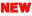Note: In recent versions of Delphi's Pascal compiler, the Round function is based on the FPU processor of the CPU. This processor adopts the so-called "Banker's Rounding", which rounds middle values (as 5.5 or 6.5) up and down depending whether they follow an odd or an even number.

## Conclusion

In this chapter we've explored the basic notion of type in Pascal. But the language has another very important feature: It allows programmers to define new custom data types, called user-defined data types. This is the topic of the next chapter.

### Next Chapter: User-Defined Data Types